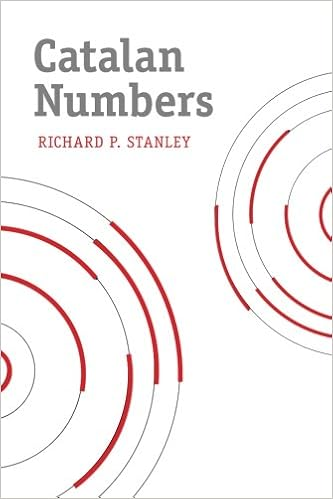Then [Dan Piponi](https://twitter.com/sigfpe/status/1015384915058176001), another one of my internet math pals, went back to the 4d case and thought about the _moments_ of the distance for 2 randomly chosen points on the unit sphere in \\(\mathbb{R}^4\\). The first moment is the mean, the second moment is the variance, and so on.

He notice that the _even_ moments were the [Catalan numbers](https://en.wikipedia.org/wiki/Catalan_number)

\$\$ 2, 5, 14, 42, 132 \dots \$\$

> Pick a pair of points uniformly randomly on the 4-sphere. Let S be the distance between them. E[S²ⁿ] is the (n+1)-th Catalan number.

This was funny, because he'd just managed to buy a hardback copy of Richard P. Stanley's book on Catalan numbers on Amazon for just 11 dollars, thanks to some glitch that quickly went away.